Year 7 Interactive Maths - Second Edition

## Drawing Angles less than 180° with a Protractor

To draw an angle with a protractor, proceed as follows:
• Draw a straight line (i.e. an arm of the angle).
• Place a dot at one end of the arm.  This dot represents the vertex of the angle.
• Place the centre of the protractor at the vertex dot and the baseline of the protractor along the arm of the angle.
• Find the required angle on the scale and then mark a small dot at the edge of the protractor.
• Join the small dot to the vertex with a ruler to form the second arm of the angle.
• Label the angle with capital letters.

#### Example 1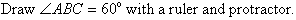##### Solution: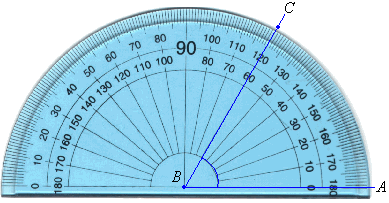• Draw a straight line AB.
• Place a dot at B.  This dot represents the vertex of the angle.
• Place the centre of the protractor at B and the baseline of the protractor along the arm BA.
• Find 60º on the scale and mark a small dot at the edge of the protractor.
• Join the vertex B to the small dot with a ruler to form the second arm, BC, of the angle.
• Mark the angle with a small arc as shown below.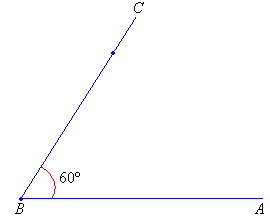## Drawing Reflex Angles

To draw a reflex angle (i.e. angle greater than 180º and less than 360º), proceed as follows:

• Subtract the reflex angle from 360º.  Then draw the resulting angle as described earlier.
• The required angle is outside the one that has been drawn.
• Mark the angle with a small arc.
• Label the angle.

#### Example 2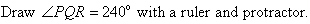##### Solution: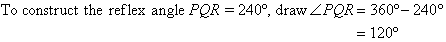• Draw a straight line PQ.
• Place a dot at Q.  This dot represents the vertex of the angle.
• Turn the protractor upside down and place the centre of the protractor at Q and the baseline of the protractor along the arm PQ.
• Use the outer scale to find 120º and mark a small dot at the edge of the protractor.• Then remove the protractor and join the vertex, Q, to the small dot with a ruler to form the second arm, QR, of the angle.
• Mark the angle with a small arc as shown below.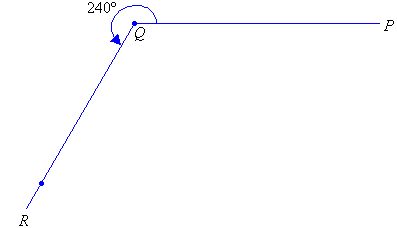### Activity 1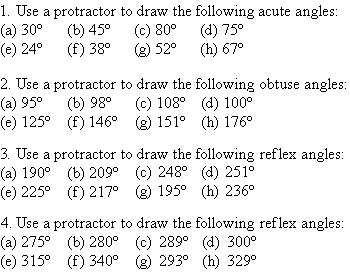Copyright © 2000-2020 mathsteacher.com Pty Ltd.  All rights reserved.
Australian Business Number 53 056 217 611

Copyright instructions for educational institutions

Please read the Terms and Conditions of Use of this Website and our Privacy and Other Policies.
If you experience difficulties when using this Website, tell us through the feedback form or by phoning the contact telephone number.